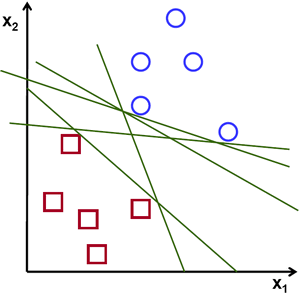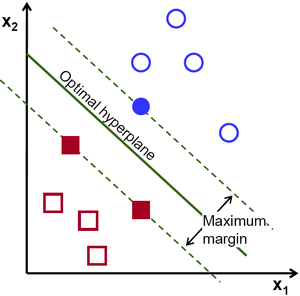# Vector

## What is a Vector?

A vector is a mathematical object that encodes a length and direction.  Conceptually they can be thought of as representing a position or even a change in some mathematical framework or space.  More formally they are elements of a vector space:  a collection of objects that is closed under an addition rule and a rule for multiplication by scalars.

A vector is often represented as a 1-dimensional array of numbers, referred to as components and is displayed either in column form or row form. Represented geometrically, vectors typically represent coordinates within a n-dimensional space, where n is the number of dimensions. A simplistic representation of a vector might be a arrow in a vector space, with an origin, direction, and magnitude (length).

## How do Vectors work?

As basic units for computational arithmetic, vectors can be transformed utilizing basic mathematics. For example, vectors can be added, subtracted and multiplied by. For instance, vector addition can be denoted as:
a + b = (a1 + b1, a2 + b2, a3 + b3)
Furthermore, several rules vector multiplication can be defined.  One such is the pointwise product and is denoted in a similar way:
a * b = (a1 * b1, a2 * b2, a3 * b3)
Additionally, a vector dot product can calculate the sum of the multiplied elements of two vectors of the same length to give a scalar:
a . b = (a1 * b1 + a2 * b2 + a3 * b3)

### Vectors and Machine Learning

Vectors are commonly used in machine learning as they lend a convenient way to organize data.  Often one of the very first steps in making a machine learning model is vectorizing the data.

They are also relied upon heavily to make up the basis for some machine learning techniques as well. One example in particular is support vector machines. A support vector machine analyzes vectors across an n-dimensional space to find the optimal hyperplane for a given data set. In essence, a support vector machine will attempt to find a line that have the maximum distance between data sets of both classes. This allows for future data points to be classified with ore confidence, due to increased reinforcement.Image Source

### Vectors vs. Scalars

A vector is a data structure with at least two components, as opposed to a scalar, which has just one. For example, a vector can represent velocity, an idea that combines speed and direction: wind velocity = (50mph, 35 degrees North East). A scalar, on the other hand, can represent something with one value like temperature or height: 50 degrees Celsius, 180 centimeters. Therefore, we can represent two-dimensional vectors as arrows on an x-y graph, with the coordinates x and y each representing one of the vector’s values.